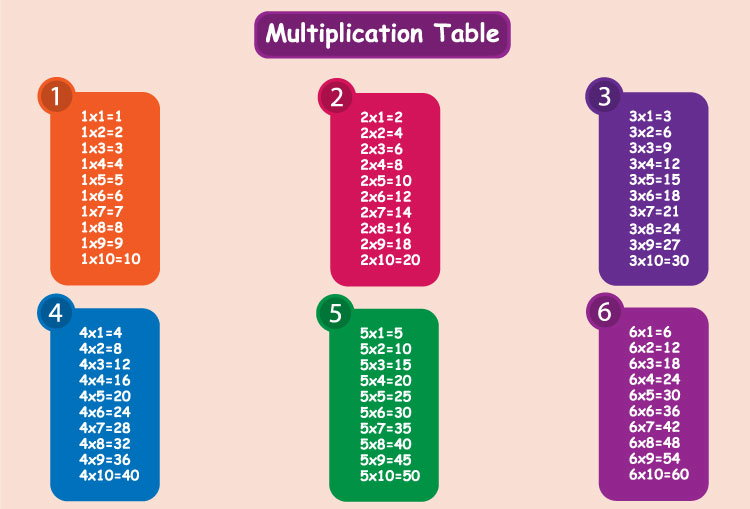# C Program to Generate Multiplication Table

Example to generate the multiplication table of a number (entered by the user) using for loop.To understand this example, you should have the knowledge of following C programming topics:

The program takes an integer input from the user and generates the multiplication table up to 10.

## Example #1: Multiplication Table Up to 10

``````#include <stdio.h>
int main()
{
int n, i;

printf("Enter an integer: ");
scanf("%d",&n);

for(i=1; i<=10; ++i)
{
printf("%d * %d = %d \n", n, i, n*i);
}

return 0;
}
``````

Output

```Enter an integer: 9
9 * 1 = 9
9 * 2 = 18
9 * 3 = 27
9 * 4 = 36
9 * 5 = 45
9 * 6 = 54
9 * 7 = 63
9 * 8 = 72
9 * 9 = 81
9 * 10 = 90
```

Here's a little modification of the above program to generate the multiplication table up to a range (where range is also a positive integer entered by the user).

## Example #2: Multiplication Table Up to a range (entered by the user)

``````#include <stdio.h>
int main()
{
int n, i, range;

printf("Enter an integer: ");
scanf("%d",&n);

printf("Enter the range: ");
scanf("%d", &range);

for(i=1; i <= range; ++i)
{
printf("%d * %d = %d \n", n, i, n*i);
}

return 0;
}
``````

Output

```Enter an integer: 12
Enter the range: 8
12 * 1 = 12
12 * 2 = 24
12 * 3 = 36
12 * 4 = 48
12 * 5 = 60
12 * 6 = 72
12 * 7 = 84
12 * 8 = 96
```